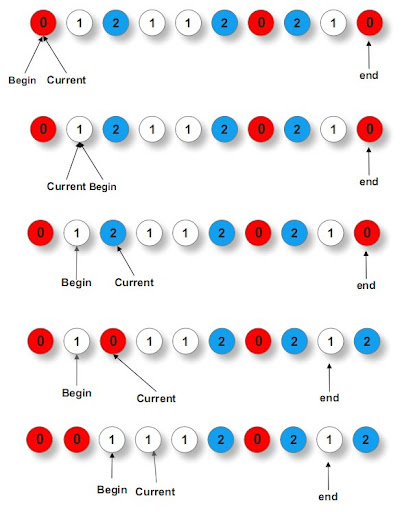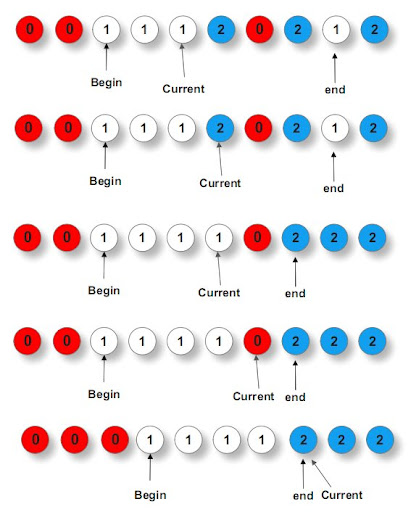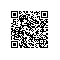# [算法系列之十一]荷兰国旗问题

#### 【思路一】

First, iterate the array counting number of 0's, 1's, and 2's, then overwrite array with total number of 0's, then 1's and followed by 2's.

（1）遍历数组，统计红白蓝三色球（0，1，2）的个数

（2）根据红白蓝三色球（0，1，2）的个数重排数组

#### 【代码一】

    /**------------------------------------
*   日期：2015-02-02
*   作者：SJF0115
*   题目: 75.Sort Colors
*   网址：https://oj.leetcode.com/problems/sort-colors/
*   结果：AC
*   来源：LeetCode
*   博客：
---------------------------------------**/
class Solution {
public:
void sortColors(int A[], int n) {
if(n <= 1){
return;
}//if
// 统计个数
int red = 0,white = 0,blue = 0;
for(int i = 0;i < n;++i){
if(A[i] == 0){
++red;
}//if
else if(A[i] == 1){
++white;
}//else
else{
++blue;
}//else
}//for
// 重新布局
for(int i = 0;i < n;++i){
if(red > 0){
A[i] = 0;
--red;
}//if
else if(white > 0){
A[i] = 1;
--white;
}//else
else{
A[i] = 2;
}
}//for
}
};


#### 【思路二】

（1）若遍历到的位置为1，则说明它一定属于中部，根据总思路，中部的我们都不动，然后current向前移动一个位置。

（2）若遍历到的位置为0，则说明它一定属于前部，于是就和begin位置进行交换，然后current向前移动一个位置，begin也向前移动一个位置（表示前边的已经都排好了）。

（3）若遍历到的位置为2，则说明它一定属于后部，于是就和end位置进行交换，由于交换完毕后current指向的可能是属于前部的，若此时current前进则会导致该位置不能被交换到前部，所以此时current不前进。而同1），end向前移动一个位置。#### 【代码二】

    /**------------------------------------
*   日期：2015-02-04
*   作者：SJF0115
*   题目: Sort Colors
*   网址：https://oj.leetcode.com/problems/sort-colors/
*   博客：
---------------------------------------**/
class Solution {
public:
void sortColors(int A[], int n) {
int begin = 0,end = n-1,cur = 0;
while(cur <= end){
if(A[cur] == 0){
swap(A[begin],A[cur]);
// 指向排序0末尾的下一个位置
++begin;
// 向前遍历
++cur;
}//if
else if(A[cur] == 1){
++cur;
}//else
else{
swap(A[end],A[cur]);
// 指向排序2开头的前一个位置
--end;
}//else
}//for
}
};


#### 【思路三】

A[i] == 2，直接插入blue

#### 【代码三】

    /**------------------------------------
*   日期：2015-02-03
*   作者：SJF0115
*   题目: 75.Sort Colors
*   网址：https://oj.leetcode.com/problems/sort-colors/
*   结果：AC
*   来源：LeetCode
*   博客：
---------------------------------------**/
class Solution {
public:
void sortColors(int A[], int n) {
if(n <= 1){
return;
}//if
int red = -1,white = -1,blue = -1;
for(int i = 0;i < n;++i){
// 插入red对white blue有影响
if(A[i] == 0){
// blue整体向后移动一位
A[++blue] = 2;
// white整体向后移动一位
A[++white] = 1;
// 插入red
A[++red] = 0;
}//if
// 插入white blue受到影响
else if(A[i] == 1){
// blue整体向后移动一位
A[++blue] = 2;
// 插入white
A[++white] = 1;
}//else
// 插入blue对其他没有影响
else{
// 插入blue
A[++blue] = 2;
}//else
}//for
}
};使用钉钉扫一扫加入圈子
+ 订阅